• 概率密度函数：NORMDIST(B2,AVERAGE(B:B),STDEV.S(B:B),0) STDEV.S：标准差 0：概率密度函数 df越大，越接近正态分布，趋于正无穷时候贴近正态分布
概率密度函数：NORMDIST(B2,AVERAGE(B:B),STDEV.S(B:B),0) STDEV.S：标准差 0：概率密度函数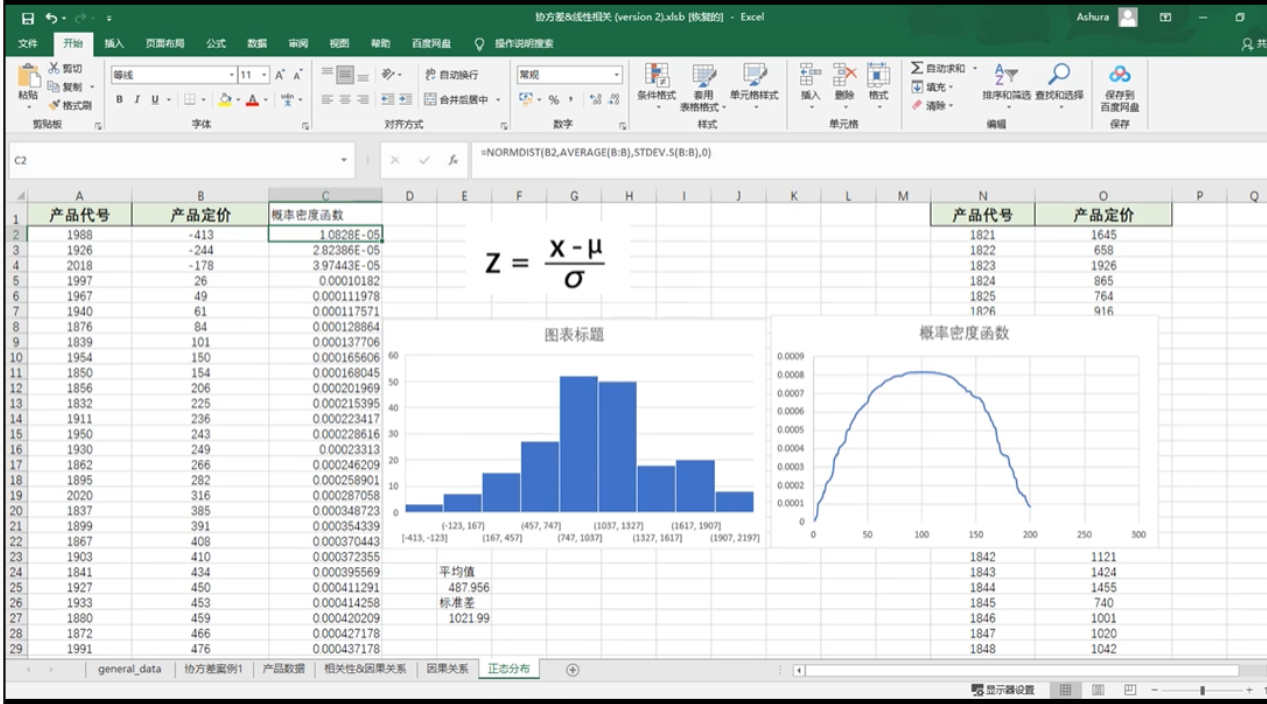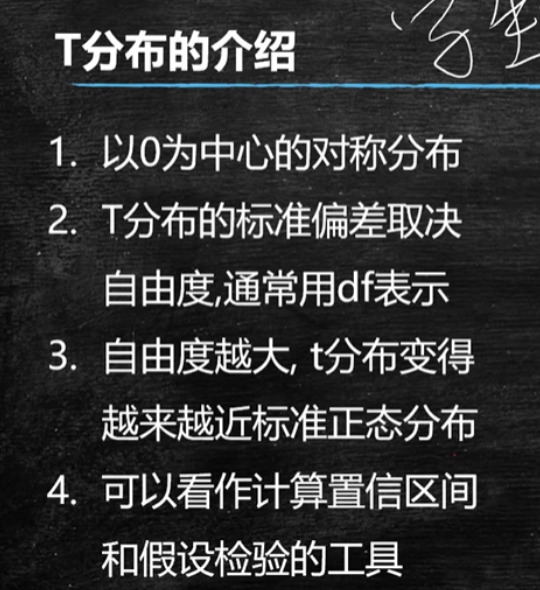df越大，越接近正态分布，趋于正无穷时候贴近正态分布。 T-DIST(value，DF，TRUE）
展开全文T分布
• 在制造业工厂为了统计产品尺寸的分布程度，需要制作正态分布图，excel 中没有专门的概率分布图制作比较麻烦。使用python容易实现。 运行环境： win10 win8 win7 64位操作系统 python 3.6 以上 第三方库： numpy ...
Python读取excel生成正态分布图
需求背景： 在制造业工厂为了统计产品尺寸的分布程度，需要制作正态分布图，excel 中没有专门的概率分布图制作比较麻烦。使用python容易实现。 运行环境： win10 win8 win7 64位操作系统 python 3.6 以上 第三方库： numpy matplotlib xlrd 程序代码:
import matplotlib.pyplot as plt
import numpy as np
import matplotlib
import matplotlib.mlab as mlab
import xlrd
#import time

def get_excel_value(j,filename):  #j代表列0是第一列,j,filename 为文件名
row_list =[] #建立空列表插入尺寸数据
excel = xlrd.open_workbook(r"%s"%filename)
#sheet = excel.sheet_by_index(0)  #根据下标获取对应的sheet表
sheet = excel.sheet_by_name("report")  #根据表名获取对应的sheet表
#for  j in range(0,sheet.ncols):
for i in range(5, sheet.nrows):     #从表的第6行开始到
if sheet.row_values(i, start_colx=j, end_colx=j+1):  #内循环
row_list.append(sheet.row_values(i, start_colx=j, end_colx=j+1)) #每次循环取一个单元格数据插入列表中
arr_mean = np.mean(row_list)
arr_std = np.std(row_list,ddof=1)
return row_list,arr_mean,arr_std   #函数返回尺寸数据,尺寸均值,尺寸标准差

def get_tolerance(j,filename):  #读取公差函数，公差在工作表的3-5行
excel = xlrd.open_workbook(r"%s"%filename)
sheet = excel.sheet_by_index(0)  #根据下标获取对应的sheet表
nominalvalue = sheet.row_values(2, start_colx=j, end_colx=j+1)
up=sheet.row_values(3, start_colx=j, end_colx=j+1)
low=sheet.row_values(4, start_colx=j, end_colx=j+1)
LSL=nominalvalue+low
USL=nominalvalue+up
return LSL,USL

def get_name(j,filename):   #获取尺寸名
excel = xlrd.open_workbook(r"%s"%filename)
sheet = excel.sheet_by_index(0)  #根据下标获取对应的sheet表
name = sheet.row_values(1, start_colx=j, end_colx=j+1)
return name

class higram:

def __init__(self,dimension,filename,mu,sigma,data,LSL,USL,num_bins ):

self.dimension=dimension
self.filename=filename
self.mu=mu
self.sigma=sigma
self.data=data
self.LSL=LSL
self.USL=USL
self.num_bins=num_bins

def creatchart(self):

# 设置matplotlib正常显示中文和负号
matplotlib.rcParams['font.sans-serif']=['SimHei']   # 用黑体显示中文
matplotlib.rcParams['axes.unicode_minus']=False     # 正常显示负号

def norm_pdf(x,mu,sigma):
pdf = np.exp(-((x - mu)**2) / (2* sigma**2)) / (sigma * np.sqrt(2*np.pi)) #正态分布函数
return pdf

n, bins, patches = plt.hist(self.data, self.num_bins, density=1, facecolor="blue", edgecolor="black", alpha=0.5)#facecolor直方填充颜色edgecolor直方边框颜色

#FFC0CB Pink 粉红 #E6E6FA Lavender 淡紫色/熏衣草淡紫

y=norm_pdf(bins, mu, sigma)   #概率分布图
#print(y)

plt.plot(bins, y, 'r--',linestyle='-')   #概率分布图"_"代表实线,"r--"代表红色

x = np.linspace(self.LSL, self.LSL, 100)    #等差数列
y = np.linspace(0, 60, 100)
plt.text(x,y,'LSL',size=13)    #text(x,y,str),x,y 文字的坐标
plt.plot(x, y, 'g--', linewidth=1)

x = np.linspace(self.USL, self.USL, 100)
y = np.linspace(0, 60, 100)
plt.text(x,y,'USL',size=13)
plt.plot(x, y, 'g--', linewidth=1)

plt.xlabel("区间")# 显示纵轴标签

plt.ylabel("频数/频率") # 显示图标题

plt.title(self.dimension+"_正态分布图")

plt.show()

if __name__ == "__main__":
#s=time.time()
data=get_excel_value(0,"检测报告.xls")  #尺寸数据
mu=get_excel_value(0,"检测报告.xls")    #尺寸均值
sigma=get_excel_value(0,"检测报告.xls")     #尺寸标准差
LSL=get_tolerance(0,"检测报告.xls")       #下公差
USL=get_tolerance(0,"检测报告.xls")       #上公差
Dimension=get_name(0,"检测报告.xls")
#尺寸名
#e=time.time()
#t=e-s
#print(t)
'''print("result:",data)
print("mean:",mu)
print("std:",sigma)
print("LSL:",LSL)
print("USL:",USL)
print("Dimension:",Dimension)'''
h=higram(Dimension,"检测报告.xls",mu,sigma,data,LSL,USL,num_bins=60)
h.creatchart()

运行效果：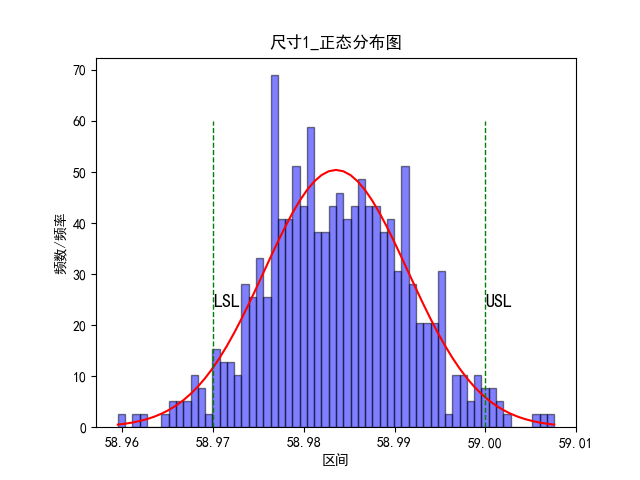展开全文python
• python对数据列表进行正态分布检验 最近刚入门python，欢迎大家多多指教！ 最近接到老师的一个项目，要求对一些医疗数据进行分析，数据类似于： 其中PROMS_SERIAL_NO是各例手术的编号，HR_SCORE_CHANGE是对手术的...
最近刚入门python，欢迎大家多多指教！ 最近接到老师的一个项目，要求对一些医疗数据进行分析
现在编写一个allfunction.py函数文件，使得获取excel的数据后，可以对数据进行数据分析。
from xlrd import open_workbook
from xlutils.copy import copy
import xlrd
import xlwt
from pandas.core.frame import DataFrame
from datetime import date, datetime
import numpy as np
import pandas as pd
import matplotlib.pyplot as plt
from scipy import stats
from xlutils.copy import copy

# 绘图大致判断其是否符合正态分布
def drawing(list,drawfilename):
c = {"HR_SCORE_CHANGE": list}
datas = DataFrame(c)
print(datas)

fig = plt.figure(figsize = (10,6))
datas.hist(bins=50,ax = ax2)
datas.plot(kind = 'kde', secondary_y=True,ax = ax2)
plt.grid()
plt.savefig(drawfilename + "描绘正态分布直方图.png")  # 保存图片
#plt.show()
plt.cla()
plt.clf()
plt.close()

#list为分析的列表，string为excel中的添加的总标题名
def analyselist(list,string):
# .kstest方法：KS检验，参数分别是：待检验的数据，检验方法（这里设置成norm正态分布），均值与标准差
# 结果返回两个值：statistic → D值，pvalue → P值,p值大于0.05，为正态分布
df = pd.DataFrame(list, columns=['value'])
u = df['value'].mean()  # 计算均值
std = df['value'].std()  # 计算标准差
result = stats.kstest(df['value'], 'norm', (u, std))

#数据记入至excel表
r_xls = open_workbook("Summary of analysis results.xls") # 读取excel文件
rows_old = r_xls.sheets().nrows # 获取已有的行数
excel = copy(r_xls) # 将xlrd的对象转化为xlwt的对象
table = excel.get_sheet(0) # 获取要操作的sheet
#print (rows_old)
#对excel表追加一行内容

table.write_merge(1 + rows_old, 1 + rows_old, 0, 6, string) #合并0至6列的单元格，写入string
title = ["最大值", "最小值", "平均值", "方差", "标准差", "变异系数", "pvalue"]
# 写入"最大值", "最小值", "平均值", "方差", "标准差", "变异系数", "pvalue"标题

for i in range(0, len(title)):
table.write(2 + rows_old, i, title[i])

table.write(3 + rows_old, 0, str(np.max(list)))
table.write(3 + rows_old, 1, str(np.min(list)))
table.write(3 + rows_old, 2, str(format(np.mean(list),'.4f')))
table.write(3 + rows_old, 3, str(format(np.var(list),'.4f')))
table.write(3 + rows_old, 4, str(format(np.std(list), '.4f')))
table.write(3 + rows_old, 5, str(format((np.std(list, ddof=1)/np.mean(list)), '.4f')))
table.write(3 + rows_old, 6, result)

if float(result)> 0.05:
table.write_merge(4 + rows_old, 4 + rows_old, 0, 6, "输出结果pvalue>0.05，接受其符合正态分布的假设！")
else:
table.write_merge(4, 4, 0, 6, "输出结果pvalue<0.05，不接受其符合正态分布的假设！")

excel.save("Summary of analysis results.xls") # 保存并覆盖文件


结果如下：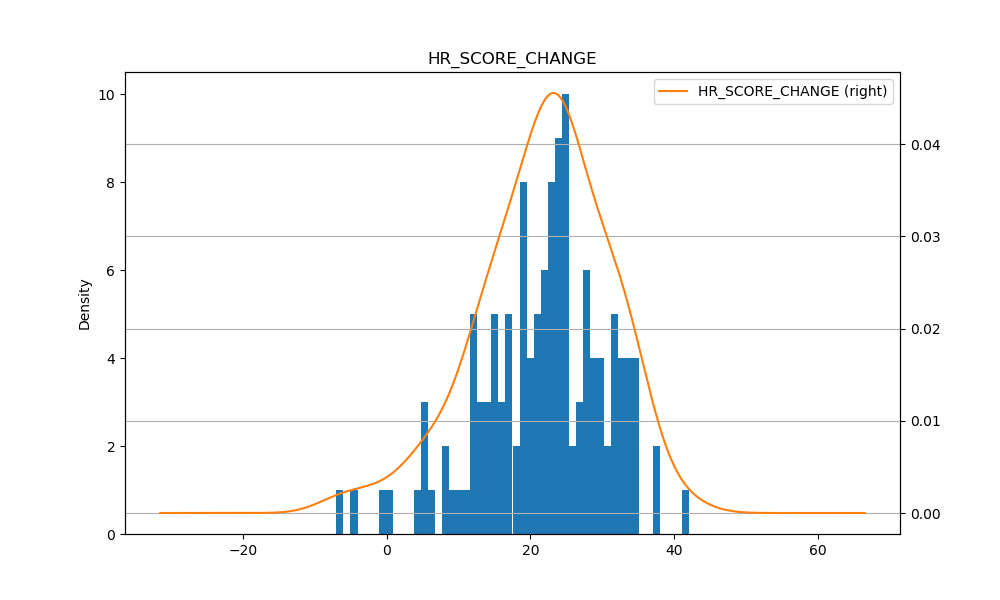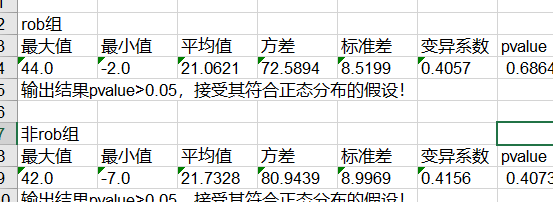代码借鉴了很多CSDN博主和博客园上发表的文章，涉及较多，没有一一记录，在这里向他们表示感谢，初学python很多东西不是特别熟练，欢迎大家多多交流 其实使用pandas写入excel更为方便 例如：要使用pandas 筛选出客户姓名以大写字母J 开头的那些行
#!/usr/bin/env python3
import pandas as pd
import sys
input_file = sys.argv
output_file = sys.argv
data_frame_value_matches_pattern = data_frame[data_frame['Customer Name'].str.startswith("J")]
writer = pd.ExcelWriter(output_file)
data_frame_value_matches_pattern.to_excel(writer, sheet_name='jan_13_output',index=False)
writer.save()

展开全文python 数据分析
• 这篇文章主要讲讲如何判断数据是否符合正态分布。主要分为两种方法：描述统计方法和统计检验方法。描述统计方法描述统计就是用描述的数字或图表来判断数据是否符合正态分布。常用的方法有Q-Q图、P-P图、直方图、茎叶...


- 点击上方
“中国统计网”
订阅我吧！-在前面的文章中讲过，很多模型的假设条件都是数据是服从正态分布的。这篇文章主要讲讲如何判断数据是否符合正态分布。主要分为两种方法：描述统计方法和统计检验方法。
描述统计方法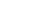描述统计就是用描述的数字或图表来判断数据是否符合正态分布。常用的方法有Q-Q图、P-P图、直方图、茎叶图。
1. Q-Q图
此Q-Q非用于聊天的QQ，Q是quantile的缩写，即分位数。分位数就是将数据从小到大排序，然后切成100份，看不同位置处的值。比如中位数，就是中间位置的值。
Q-Q图的x轴为分位数，y轴为分位数对应的样本值。x-y是散点图的形式，通过散点图可以拟合出一条直线，如果这条直线是从左下角到右上角的一条直线，则可以判断数据符合正态分布，否则则不可以。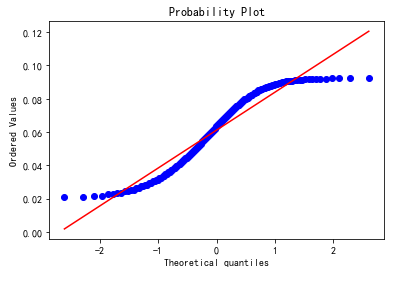拟合出来的这条直线和正态分布之间有什么关系呢？为什么可以根据这条直线来判断数据是否符合正态分布呢。
我们先来想一下正态分布的特征，正态分布的x轴为样本值，从左到右x是逐渐增大的，y轴是每个样本值对应的出现的概率。概率值先上升后下降，且在中间位置达到最高。
可以把Q-Q图中的y轴理解成正态分布中的x轴，如果拟合出来的直线是45度，可以保证中位数两边的数值分布是一样的，即正态分布中基于中位数左右对称。
在Python中可以使用如下代码来绘制Q-Q图：
from scipy import statsfig = plt.figure()res = stats.probplot(x, plot=plt)plt.show()
与Q-Q图类似的是P-P图，两者的区别是前者的y轴是具体的分位数对应的样本值，而后者是累计概率。
2. 直方图
直方图分为两种，一种是频率分布直方图，一种是频数分布直方图。频数就是样本值出现的次数，频率是某个值出现的次数与所有样本值出现总次数的比值。
在Python中我们可以使用如下代码来绘制频数分布直方图：
import matplotlib.pyplot as pltplt.hist(x,bins = 10)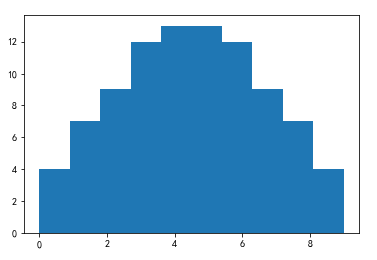可以使用如下代码来绘制频率分布直方图：
import seaborn as snssns.distplot(x)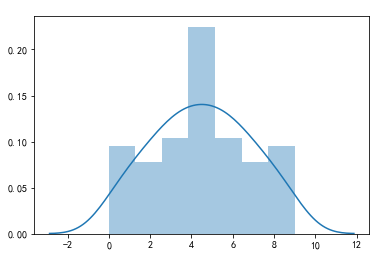与直方图类似的还有茎叶图，茎叶图是类似于表格形式去表示每个值出现的频次。
统计检验方法SW检验中的S就是偏度，W就是峰度，峰度和偏度与正态的关系我们在前面的文章有讲过，没看过的同学可以去看看：你到底偏哪边的？
1. KS检验
KS检验是基于样本累积分布函数来进行判断的。可以用于判断某个样本集是否符合某个已知分布，也可以用于检验两个样本之间的显著性差异。
如果是判断某个样本是否符合某个已知分布，比如正态分布，则需要先计算出标准正态分布的累计分布函数，然后在计算样本集的累计分布函数。两个函数之间在不同的取值处会有不同的差值。我们只需要找出来差值最大的那个点D。然后基于样本集的样本数和显著性水平找到差值边界值(类似于t检验的边界值)。判断边界值和D的关系，如果D小于边界值，则可以认为样本的分布符合已知分布，否则不可以。

PDF( probability density function)：概率密度函数CDF( cumulative distribution function)：累积分布函数，是概率密度函数的积分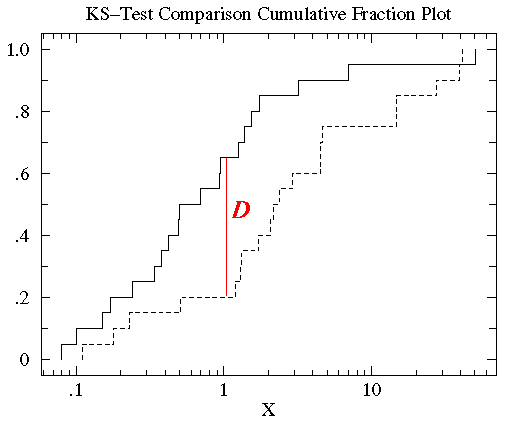在Python中有现成的包可以直接用于KS检验：

from scipy.stats import kstestkstest(x,cdf = "norm")
x表示待检验的样本集，cdf用来指明要判断的已知分布类型，有：‘norm’,’expon’,’logistic’,’gumbel’,’gumbel_l’, gumbel_r’,‘extreme1’值可以选，其中norm表示正态分布检验。
kstest会返回两个值：D和对应的p_value值。
在Python中可以用如下代码：

from scipy.stats import andersonanderson(x, dist='norm')
x为待检验的样本集，dist用来指明已知分布的类型。可选值与ks检验中可选值一致。
上面代码会返回三个结果： 第一个为统计值，第二个为评判值，第三个为每个评判值对应的显著性水平
3. W检验
W检验(Shapiro-Wilk的简称)是基于两个分布的相关性来进行判断，会得出一个类似于皮尔逊相关系数的值。值越大，说明两个分布越相关，越符合某个分布。
在Python中的实现代码如下：

from scipy.stats import shapiroshapiro(x)
上面的代码会返回两个结果：W值和其对应的p_value。
shapiro是专门用于正态性检验的，所以不需要指明分布类型。且 shapiro 不适合做样本数＞5000的正态性检验。
非正态数据的处理办法一般数据不是正态就是偏态，如果偏态不严重可以对数据取平方根来进行转换。如果偏态很严重，则可以对数据进行对数转换。转换方法在偏态文章中也有讲过。

End.
来源：俊红的数据分析之路

· 零基础入职数据分析就业班 ·
课程形式主为“直播+录播”
课程专享：月考测试通关+课程项目作业+1v1职场生涯规划+班主任辅导学习+资深讲师答疑
课程结束后能熟练掌握SQL、Python、Excel、PPT等数据分析工具
金3银4招聘季，爱数据带你成功入职数据分析！点击“阅读原文”入职数据分析


展开全文•   在进行数据分析时，为了直观显示成绩分布规律，即是正态，正偏态和负偏态三种分布状态，可以画总分及各学科的正态分布图，其作用一是对学生进行比较，通过分布曲线，可以分析出学生成绩是不是存在两极分化（两头...数据分析 统计学
• 正态分布广泛存在于自然现象、生产、生活的方方面面，例如试卷命题难度，产品的使用寿命、农作物产量、气温、降水量、工资收入、人类的身高体重肺活量，甚至颜值……关于正态分布的数学定义及各种性质，不在此赘述。...
• ↑ 点击上方“可乐的数据分析之路”关注 + 星标~大概率每天早8点25更新哈喽，大家好，我是可乐今天这篇文章接2个月以前的那篇文章离散型随机变量的概率分布，继续来聊聊连续型随...统计学 数据分析 twitter 数据可视化
• 正态分布的检验方法有很多，我们在<<正态分布与方差齐性的检验方法与SPSS操作>>做过介绍，本文介绍的R软件的检验。每种方法在R中都有很多程序包可以实现。 示例采用<<完全随机资料设计的方差分析...R语言 编程语言 大数据
• SPSS如何验证是否符合正态分布 正态分布是T检验等统计分析的前提交通，本经验将介绍如何使用SPSS验证是否符合正态分布。 工具/原料 SPSS Statistics 测试数据集 方法/步骤 1 首先准备测试数据集，...
• ##通过分位数-分位数图来验证收益率是否符合正态分布 import statsmodels.api as sm fig, axes = plt.subplots(3,2,figsize=(10,12)) for i in range(0,3): for j in range(0,2): sm.qqplot(log_returns.iloc[:,2*...
• 多元正态分布的检验多元正态分布mshapiro.test {mvnormtest}mvn {MVN} 多元正态分布 转自个人微信公众号【Memo_Cleon】的统计学习笔记：多元正态分布检验的R实现方法。 多元正态分布也称多元高斯分布。如同正态分布...数据分析 统计学 r语言
• 几种分布中：其中对数正态分布在统计分析中运用最为广泛，顾名思义，这种类型的分布在取对数之后服从正态分布。因为其具有这样的良好属性，在精确度要求并不严格的统计分析中。描述性统计分析 – 直接正态分布描述就...数据分析 假设检验 统计学
• pd.read_excel(path, sheet_name='two-tailed') critical_value = critical_values.loc[critical_values['n']==n][dection_level] critical_value = float(critical_value....python
• 正态分布（或者近似正态分布）是诸多参数检验的前提条件，没有这个前提，后续的判断也就没有了意义。正如你打算找一个女人做老婆，你可以通过各种描述、推断她的样子，但前提是她得是一个女人，如果不是女人甚至不是...
• 2.3 概率统计中的正态分布和偏态分布 概率可以理解为随机出现的相对数。随机现象是相对于决定性现象而言的。在一定条件下必然发生某一结果的现象称为决定性现象。随机现象则是指在基本条件不...
• ###正态分布的K-S Test 思路就是先假设原数据符合正态分布，算出原始数据的实际分布特征，然后算出理论分布特征，设置一个置信区间，根据样本数量得出D值，与理论分布和实际分布的最大离差相比较，若最大离差小于D...java matlab python
• .kstest方法：KS检验，参数分别是：待检验的数据，检验方法（这里设置成norm正态分布），均值与标准差 # 结果返回两个值：statistic → D值，pvalue → P值 # p值大于0.05，为正态分布 df['value'].plot(kind='kde',...
• Q-Q图 Q-Q图是一种散点图,对应于正态分布的Q-Q图,就是由标准正态分布的分位数为横坐标,样本值为纵坐标的散点图. 要利用QQ图鉴别样本数据是否近似于正态分布,只需看QQ图上的点是否近似地在一条直线附近,而且该直线的...
• 首先，正态分布是最重要的一种概率分布，正态分布（Normal distribution)，也称高斯分布（Gaussian distribution），具体详细的介绍可自行网上查阅资料； 其次，如下图中所示的：分位数、中位数、众数等； 再者...大数据
• Q-Q图是检验任何随机变量（如正态分布、指数分布、对数正态分布等）分布的图形方法，是观察任何分布性质的一种统计方法。 例如，如果给定的一个分布需要验证它是否是正态分布，我们运行统计分析并将未知分布与已知...
• 对于上侧检验，当 b s > b s ( n ) b_s > b_s(n) bs​>bs​(n) 时，则判断 x ( n ) x_{(n)} x(n)​ 为歧离值，所谓歧离值，就是有概率会成为离群值的数。之后，再择定一个更为严格的剔除水平，再次根据表或插值法，...
• # 判断数据是否属于正态分布 if n < 4: raise(Exception('Epps-Pulley 检验使用时，数据量要在 4 个及以上才能获得精确结果')) elif n < 10: # 若 样本量 小于 10 个，则可以直接从...python
• 用SPSS对个股收益率的正态性进行检验（超详细）数据的获取将Excel文件导入到SPSS中正态性检验方法图示法直方图P-P图Q-Q图计算法偏度和峰度Shapiro - Wilk检验和Kolmogorov - Smirnov检验结论 网上的很多教程讲的比较...
• (1)、如果数据服从正态分布，在3σ原则下，异常值被定义为与平均值偏差超过3倍标准差的值。 在正态分布情况下，距离平均值3α之外的值出现的概率为 P(|x-μ|>3σ) ≤ 0.003，属于极个别的小概率事...
• ## 如何检验数据样本的正态性？

万次阅读 多人点赞 2018-09-18 00:15:36
正态分布是自然界中最常见的也是一种最重要的分布。因此，人们在使用统计分析方法时，总是乐于正态假定，但该假定是否成立，就需要进行正态性检验了。 定义： 指如果一组观测值来自正态总体.具有正态分布的特性，...统计学
• 对Xi参数的实际值做一个假设，然后在这个假设成立的情况下，利用已知的样本信息构造一个符合一定分布的（如正态分布、T分布和F分布）的统计量，然后从理论上计算得到这个统计量的概率，如果概率很低（5%以下），根据...回归分析
• 描述正态分布（近似正态分布）资料的 频数分布 标准误 样本均数的变异程度，表示抽样误差的大 小。标准误差越大表示抽样误差越大，样本 均数的可靠性越小；标准误越小表示抽样 误差越小，样本均数的可靠性越...假设检验 统计学...